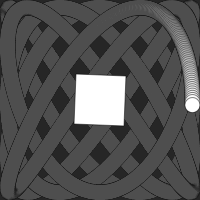# p5.layersp5.layers is a p5.js library that simplifies some common use cases for p5.js Graphics objects.

It does this by addings this functionality to p5.js:

• A new pair of functions, `beginLayer()` and `endLayer()`, handles variable-free creation and use of a Graphics.
• The p5.js draw and other canvas functions (e.g. `rect()` , `fill()`) operate either on the canvas, or on the current layer (p5.Graphics instance), depending on whether they are called between a call to `beginLayer()` / `endLayer()`.You can find a collection of examples here.

For example:

``````// with p5.layer
function draw() {
// ...
beginLayer();
background(100);
fill('blue');
circle(width / 2, height / 2, 100);
endLayer();
}
``````

is equivalent to:

``````// without p5.layer
function setup() {
// ...
let pg = createGraphics(100, 100);
}

function draw() {
// ...
pg.background(100);
pg.fill('blue');
pg.circle(pg.width / 2, pg.height / 2, 100);
image(pg, 0, 0);
}
``````

The version with `beginLayer()` doesn't require the use of `pg.` as a prefix in order to affect the created Graphics. This makes it easier to change your mind about what goes inside of a layer (or what happens in which of several layers), and to write functions that can apply to either the canvas and or a layer.

The Graphics instances that `beginLayer()` creates persist across calls to `draw()`. For example, the following code, from examples/lissajous/sketch.js, leaves a trail of circles, because the layer is only partially erased (the second argument to `background()` is a value that indicates partial opacity). It is drawn onto a canvas that is completely erased each frame, as required by the other code in the `draw()` function in that file.

``````  beginLayer();
background(100, 10);
let x = map(sin(millis() / 500), -1, 1, 0, width);
let y = map(sin(millis() / 700), -1, 1, 0, height);
circle(x, y, 20);
endLayer();
``````

The equivalent functionality, without using `beginLayer()` and `endLayer()`, would require code that is distributed among `setup()`, `draw()`, and the global context:

``````let pg;

function setup() {
// ...
pg = createGraphics(width, height);
}

function draw() {
// ...
pg.background(100, 10);
let x = map(sin(millis() / 500), -1, 1, 0, pg.width);
let y = map(sin(millis() / 700), -1, 1, 0, pg.height);
pg.circle(x, y, 20);
image(pg, 0, 0);
}
``````

## Installation Options

### Option 1: Using a CDN

Use the online version by adding the following line to your HTML document:

``````<script src="https://unpkg.com/p5.layers@1"></script>
``````

Alternatively, download `p5.layers.min.js` from this repository. Include it in your HTML document by adding this line, after the line that includes `p5.js` or `p5.min.js`:

``````<script src="p5.layers.min.js"></script>
``````

### Option 3: Using p5-server

The p5-server command-line tool, and the P5 Server Visual Studio Code Extension, will each infer this library from the presence of call to `beginLayer()` in a JavaScript-only sketch (one without an HTML file).

## Reference

### `beginLayer()`

Sets the p5.js global functions `background()`, `draw()` etc. to render into the specified layer. If no layer is specified, one is created.

Syntax:

`beginLayer()` `beginLayer([width, height, [renderer]])`

The first time this function is called, it creates an instance of p5.Graphics. Subsequent calls return this instance. (If the arguments are omitted, the Graphics is created at the canvas width and height.) On subsequent calls, the `width`, `height`, and `renderer` arguments are ignored, and the previously-created instance is used.

`beginLayer()` returns the Graphics.

`beginLayer(key, [width, height, renderer])`

This form can be used to create multiple layers. `beginLayer()` will create a new layer for each distinct `key`. The special key value `"new"` always creates a new layer.

`beginLayer(graphics)`

This form can be used to set global draw functions to render onto a Graphics that was created by `createGraphics()`.

### `endLayer()`

Restores the global draw functions `rect()` etc. so that they operate on the canvas again, instead of the Graphics created by or passed as an argument to `beginLayer()`. This function also draws the Graphics onto the canvas – although this behavior can be suppressed.

Syntax:

`endLayer()`

Restores the global draw functions, and draws the Graphics onto the canvas unless the argument to `enterGraphics()` was a Graphics.

`endLayer(x, y, [width, height])`

Restores the global draw functions, and draws the Graphics onto the canvas.

`endLayer(false)`

Restores the global draw functions. Does not draw the Graphics onto the canvas.

## Motivation

1. My students in Creative Coding frequently wanted to use the equivalent of the Layers concept that is common in GUI painting and drawing programs. I found that this was a significant hurdle, both because of the terminology and the mechanics.
2. Because the p5.Graphics functions are methods on the instance, changing code to operate on the canvas such that it instead operates p5.Graphics requires a significant amount of editing.

## Caveats

• The code only works in global mode. It does not check whether it is running in global mode.
• The code does not validate its arguments, and does not use the p5.js Friendly Error System.
• The code has only been tested in modern browsers.

## Keeping in Touch

Report bugs, features requests, and suggestions here, or message me on Twitter.